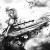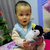## 如何用python用私钥给报文rsa加密

PrivateKey pKey = (PrivateKey)store.getKey(alias, pwd.toCharArray());
cipher.init(Cipher.ENCRYPT_MODE, pKey);

python:

with open(UNIONPAY_PRIVATE_KEY_FILE) as key_file:

msg8 = msg.encode('utf-8')
msg_dis = md5(msg8).digest()
print rsa.encrypt(msg_dis,key2)
print b64encode(rsa.encrypt(msg_dis,key2))

0rsa的两个密钥地位实际上是对等的，用一个加密用另一个就能解密

01、对方只会用你的公钥（或他的公钥）加密，所谓公钥就是你要公布给别人用的。他不会有你的私钥，因为这个私钥只有你有

2、签名算法实际上是加了一些东西的。

``````def build_rsasign(content):
private = os.path.join(cfile.KEY_PATH, 'private')
signer = PKCS1_v1_5.new(key)
d = SHA.new()
d.update(content)
sign = signer.sign(d)
signature = base64.b64encode(sign)

return signature

def check_rsasign(content, sign, stype=0):

p = 'public'
if stype == 1:
p = 'public_fuwu'

#    content = content.encode(charset)
sign = base64.b64decode(sign)
public = os.path.join(cfile.KEY_PATH, p)